## Tribology: An Introduction

Definition: The word tribology is derived from the Greek word tribos, meaning rubbing ("to rub"); thus, a direct translation of "tribology" is "the science of rubbing". However, now tribology incorporates also the studies of adhesion, contact formation, wear, fracture, lubrication, nanoindentation and, first of all, friction.

Historical remarks: The macroscopic laws of the classical (macroscopic) friction were found by da Vinci, Amontons, Euler and Coulomb.

• Leonardo da Vinci (1452-1519) made experiments on an inclined plane. He found that friction is independent on the area of contact.

The law of Leonardo appears quite bizarre: Intuitively one would expect the friction force to be proportional to the area of contact. The paradox was resolved by Bowden and Tabor (~1950, see below), distinguishing between the real area of contact and the geometric (visible) area of contact. The real area of contact is only a small fraction of the visible area of contact.

• Guillaume Amontons (1663-1705) have done experiments on a horizontal surface and measured the friction force with a spring. He observed that if double or triple the weight of the log, the friction is also doubled or tripled respectively. Thus, friction is proportional to the normal force (load) (and independent on the area of contact). Amontons called the proportional factor friction constant.

• Leonard Euler (1707-1783) pointed that one has to distinguish between the static and kinetic friction: da Vinci tested the static friction, while Amontons dealt with the kinetic friction.

• Charles Coulomb (1736-1806) measured the kinetic friction for different speeds and found that friction is independent on the velocity.

### Why friction is important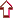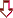• In one type of problems we have to maximize friction, for example,

(a) at ancient times (200,000 BC) frictional heating in the lighting of fires by rubbing wood on wood;

(b) nowadays pins (bolts etc., the static friction) or braking (kinetic friction between the car tires and the road).

• More often the most important is to lower friction, for example,

(a) Egyptians (2,400 BC) used water to lubricate wooden sledges used to transport large stones to build the pyramids;

(b) nowadays in machines, e.g., car engines. An example (from Persson's book): in USA friction takes away about 6% of the gross national product

### Hydrodynamic and boundary lubrication• Hydrodynamic (fluid) lubrication (Reynolds 1886) for a (macroscopically) thick liquid layer (e.g., 0.01 mm)

• use Navier-Stokes equations with appropriate boundary conditions (typically stick = locked);

• the friction is determined mainly by the viscosity of the liquid lubricant;

• an example (from Persson's book): Which lubricant is better oil (with high viscosity) or water? A drop of water between clean hands does not make the hands slip easily over each other, while a drop of oil does. Why?  The fluid is squeezed out from the contact area, but this process will take a long time if the viscosity is large.

• Boundary lubrication for a thin (few Angström) lubricant film, e.g., if the lubricant was squeezed out from the contact area

• the friction is typically much higher, e.g., by a factor of 102,  as compared with the hydrodynamic lubrication;

• the friction mainly depends not on lubricant viscosity but on the chemical composition of the fluid; usually the better lubricant corresponds to a fluid that is adsorbed by the solid substrates.

At stop/start, (almost) always the regime of boundary lubrication occurs. In what follows we consider the boundary lubrication only.

### Experimental techniques(1) Standard technique (Bowden and Tabor 1950; Rabinowicz 1965; surface forces apparatus by Bhusham, Israelachvili, Landman, Granick; experiments with paper on paper by Heslot et al)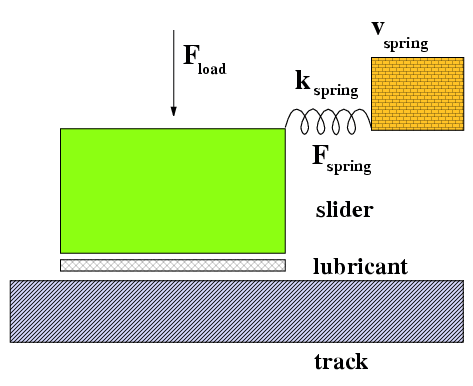typically in air the solids may be well defined as, e.g., for atomically flat mica plates the separation between the surfaces may be controlled using optical interferometry (it can be measured to within ~1Å by studying multiple beam interference finger) measured: spring force ("tribological" friction  μ ≡ Ffriction/Fload) control parameters: vspring (pulling velocity) kspring (machine stiffness) Fload (loading mass) T  (temperature)

(2) STM/AFM/FFM: scanning tunneling microscope (Gerd Binnig & Heinrich Rohrer 1981, Nobel Price 1986) for conducting surfaces, and atomic-force microscope (Binnig et al 1985) for dielectric surfaces measure surface topography;  friction-force microscope (Mate et al 1987) measures forces transverse to the surface

• can be done in ultrahigh vacuum
• typical tip radius is 10 nm to 100 nm
• typical  Fload ~ 10 nN  to 150 nN
• typical measured frictional forces F are less than or about 10–11 N
• typically operates at low velocities (v ~ 1 nm/s to 1 μm/s)
(3) Quartz-crystal microbalance (QCM) technique (Krim and Widom 1986): Gas atoms, such as Kr, Xe, or Ar, condense onto the surface of a quartz-crystal oscillator covered by a (111) oriented noble-metal substrate such as Au or Ag. The added mass of the adsorbate and the dissipation due to slip of the layer over the substrate shift and broaden the microbalance resonance peak. By measuring these changing, information about the form and magnitude of the frictional force can be obtained
• can be done in ultrahigh vacuum
• typically for one-two adsorbed layers only

(4) Levitating tribometer (O.A. Marchenko & V.S. Kulik, Institute of Physics NASU; see movie)up to submonolayer lubricant films both surfaces and film structure may be controlled with STM/AFM before and after measurments! low loads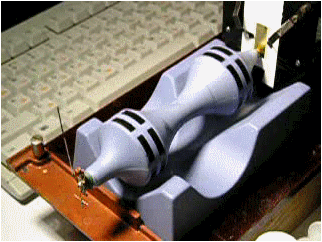(5) Molecular dynamics (MD)

• atomic-scale; atomic trajectories
• competition "size ↔ time"
• too high velocities (typically v > 1 m/s)

### Results and laws for solid-on-solid friction1. μ Ffriction/Fload ≈ constant, and  μ  is less than or about of 1 (Amontons 1699) →  real (actual) contact area  Areal~Fload  (Philip Bowden & David Tabor 1950). Indeed, real surfaces are rough. Thus,  Areal  will grow until the external loading force will be balanced by the contact pressure integrated over  Areal.

Let  Pload(real) = PloadA/Areal  be the real pressure at the contact. Then:

• at low  Pload(real) < Pyield  (elastic regime) the number of contacts increases with load

• at high  Pload(real) > Pyield  (plastic regime) the area of one contact increases (linearly) with load

2. μkineticor << μstatic,  and  μkinetic  is approximately independent of the driving velocity  v

3. Stick-slip motion ↔ smooth sliding

• stick-slip motion for soft systems and/or low velocities

• smooth sliding for stiff machines and/or high velocities

4. "Memory/age" effects: frictional forces depend on the previous dynamical history of the solid-solid contact, e.g.,  μstatic(t) ≈ as + bs ln(t)  and  μkinetic(v) ≈ μstatic(aφ/v)  with the characteristic length  aφ~1 μm  (due to plasticity of the system)

5. Full (large-scale) MD simulation showed that the lubricant structure may be

• liquid (low kinetic friction,  fstatic=0)

• amorphous (high frictions)

• solid (may be very low kinetic friction = superlubricity)

### Important notes (what is known already)1. Contacts / junctions / asperities:

• areas of real atomic contact between the two solids are small

• the contacts are randomly distributed in space over the area of apparent contact

• a typical contact size is 1 to 10 μm (may be measured optically)

An example (from Persson's book): Put a steel cube of 10 × 10 × 10 cm3  on a steel table. Taking  Pload(real) ~ Pyield ≈ 109 N/m2,  we obtain  Areal~ 0.1 mm2,  so that ~103 to 105 junctions are expected at the interface. Note: STM/AFM/FFM techniques just study a single contact.

2. Forces are of atomic-scale value close to the plasticity threshold. Estimation:

• a force per atom is  f ~ 1 eV / 1 Å = 10–19 J / 10–10 m = 10–9 N

• STM/AFM/FFM: area of one contact is  A ~ 3 Å2;  taking  Pyield ≈ 0.2 GPa (gold) to Pyield ≈ 100 GPa (diamond),  we obtain  F ~ PyieldA ~ 6·10–12 N to 3·10–9 N

• the hardness of the contacts is (much) larger than that of the material itself, because there are no dislocations in the (very small) stressed volume

• thus, (almost) always a deformation (either elastic or plastic) occurs at the contacts; this also explains Amontons law (Bowden and Tabor 1950):   μ ~ Fyield (shear)/F yield (plastic) ~ 1 and is independent on the surface area.

3. (Almost) always there is a  lubricant between the solids (called "third bodies" by tribologists): either a (specially chosen) lubricant film, or grease (oil), or dust, or wear debris produced by sliding, or water or/and a thin layer of hydrocarbon etc. adsorbed from air. Thus, the frictional force is almost entirely determined by the force required to shear the lubricant film itself.

4. (Almost) always the lubricant and the solids are "incommensurate":

• the lattice constants do not coincide in a general case

• the angles: the direction of sliding is generally at some angle to the surface lattice, and

the two surface lattices are not generally in registry

• but the situation is not so trivial often the lubricant atoms are "glued" (grafted) to the substrates (to avoid the flow of the lubricant out from the contact region), thus in fact we typically have an interface between the "glued" lubricant layer and other lubricant layers, thus the interface may be commensurate.

5. A thin film (less than 10 molecular diameters) is (almost) always layered, because the plates induce crystalline order in the film (solidify / freeze the lubricant; Thompson et al 1995). Moreover, when the lubricant thickness is less than three layers, most films behave like solid (glass-like in most cases, although may be ordered for relatively simple lubricant molecules).

See also an interesting tutorial with movies at Nano-World - a Swiss Virtual Campus Project

### Macroscopic theory (Bowder & Tabor 1950)Assume that the yield stress τs at the contact is linearly coupled with the local pressure p,

 τs(p) = τ0 + αp.

Then, integrating over the area of real contact, we obtain

and

 μ = α + τ0/

,

where <p> = Fload/Areal. Amontons' law operates if either  <p> >> τ0, or if  <p>  is constant, the latter holds for the ideally plastic surfaces (when <p> = Pyield) as well as for elastic surfaces (Volmer and Natterman 1997: real surfaces are self-affine fractals, thus as  Fload  increase, the old contacts are expanding and new contacts are created). Finally, the real area of contact must become equal to the apparent area, and Amontons' law will not operate. It is so for rubber but not for steel a machine would be destroyed earlier.

### Stick-slip motion and smooth slidingIn a typical experiment as well as in real machines, a spring is attached to the slider (even if there is no spring, the elasticity of the block plays the same role). The free end of the spring moves with a velocity vspring, and the force in the spring is registered.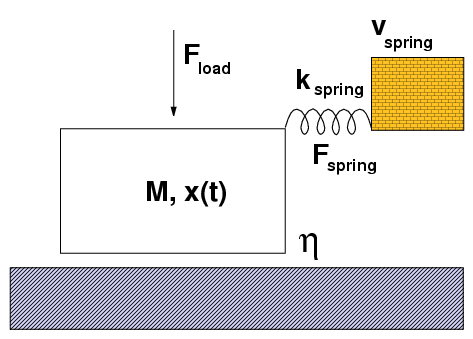Let initially the system is in rest and the spring has its natural length. Then when the base moves, the spring stretches,  f  increases until the threshold value  fs (the static frictional force), and the block starts to move. Then due to inertia, the slider accelerates to catch the base. If  vspring  is small,  f decreases until the "backward" threshold force  fb,  and the slider stops. Then the process repeats, so the stick-slip motion occurs. Otherwise, when  vspring is large, the smooth sliding takes place.
 low velocity &/or soft system                high velocity &/or stiff system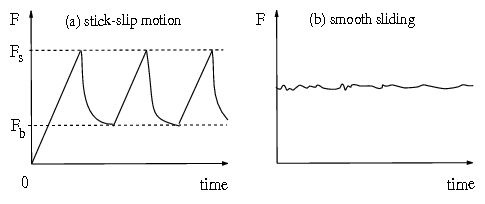Experiment: the behavior depends on the values  vspring  and  kspring. The smooth sliding is observed if the spring is stiff enough, and/or if the velocity is high enough. Otherwise, the stick-slip motion is observed. During stick, the elastic energy is pumped into the system by the driving device; during slip, this elastic energy is released into kinetic energy, and eventually dissipated as heat.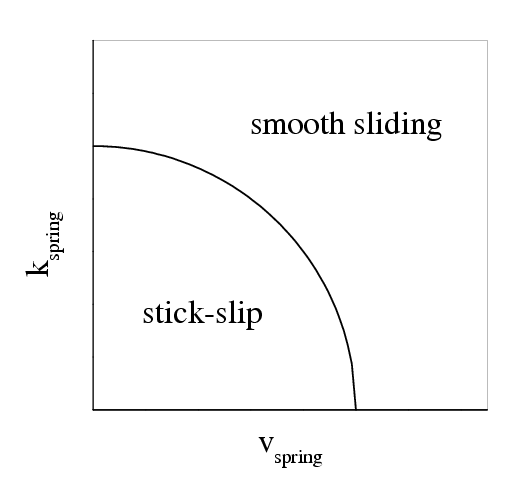Note: if one assumes that the frictional force depends on the instantaneous velocity only,  ffric= f(v),  then the boundary separating two regimes corresponds to the vertical line in the (vspring, kspring)  plane (i.e., the critical velocity is independent of kspring), that is wrong. Thus, "memory" effects must be included.

(a nice applet written by F.-J. Elmer clearly demonstrates stick-slip and smooth motion)

#### Phenomenological theoryThe phenomenological model of the transition "stick-slip motion « smooth sliding" has been developed by Heslot et al 1994, Baumberger et al 1994, and Persson 1997. The model is based on the assumption that the static frictional force depends on the time of stationary contact. For example, if the sliding-to-locked transition has occur at  t=0, then the static frictional force will evolve as

 fs(t) = fs1 + (fs2–fs1)(1–e–t/τ),
(*)
so that just after the locking the force is  fs(0) = fs1,  but later, at t, it approaches the value  fs() = fs2 > fs1.
 Qualitatively (Persson): In case (a) the spring force increases faster with  t  than the initial linear increase of the static frictional force; hence the motion of the slider will not stop and no stick-slip motion will occur; In case (b) the spring force will be smaller than the static frictional force until  t  reaches the value  t1 at which time slip starts; hence stick-slip motion will occur. Thus, the critical velocity is determined by the initial slope of the dependence  fs(t), vc ~ kspring–1 dfs(t)/dt|t=0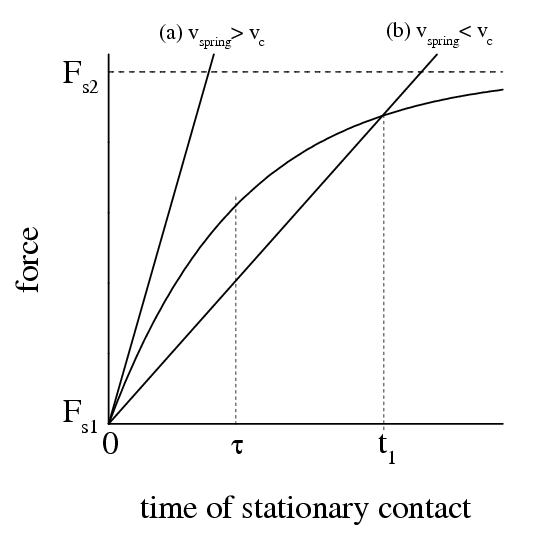The phenomenological model includes two differential equations: The motion of the top block is described by the equation
 M .. x (t) + M η . x (t) + ffric[x(t)] = fdrive[x(t)] = kspring [vspringt – x(t)],
where x(t) is the coordinate of the sliding block, M is its mass, and η is a phenomenological coefficient describing the viscous damping when the block slides over the bottom block. The second equation has to describe the frictional force ffric[x(t)]. The idea is to introduce some artificial variable called the "contact-age function" φ(t) which depends on the prehistory of the system, and is defined by the following differential equation,
 .  φ (t) = 1 – .  x (t) φ(t)/aφ
where  aφ is some characteristic distance of microscopic-scale order, e.g., the substrate lattice constant  a. Then one assumes that  ffric[x(t)]  in equation for the top block is determined by Eq.(*) where, however, instead of time one has to substitute the contact-age function  φ,  instead of  fs1,  the backward force  fb,  and instead of  fs2,  the static frictional force  fs,  so that
 ffric[x(t)] = fb + (fs– fb)( 1 – exp{–φ[x(t)]/τ} ).
(**)
Thus for the stationary contact we have φ(t) = t and we recover the dependence Eq.(*), while for the steady sliding regime  φ = aφ /vspring= τv(vspring)  does not depend on time (τv is the average time a junction survives before being broken by the sliding motion), and the force  ffric(v) = fb + (fs fb)[1 – exp(–aφ/vspringτ)]  is the constant dependent on vspring,  it is large at low velocities,  ffric= fs  for  vspring→0, and small at high velocities,  ffric= fb  for  vspring. This set of equations leads to smooth sliding for large  vspring  and to stick-slip motion at low velocities. Using the parameters Mkspring  and  vspring  corresponded to an experimental setup, taking some reasonable values for the forces  fb  and  fs,  and playing with the phenomenological parameters  τη  and  aφ,  one can achieve excellent agreement with experimental results. However, while the dependence Eq.(*) can be explained with physical models, the phenomenological dependence Eq.(**) has no good physical background, it does not follow from simulation.

### Low-dimensional modelsZero-dimensional model one atom in the periodic substrate potential: the rigid bottom substrate (external sinusoidal potential) + one atom + the dc force is applied directly to the atom + Langevin motion equation + underdamped external friction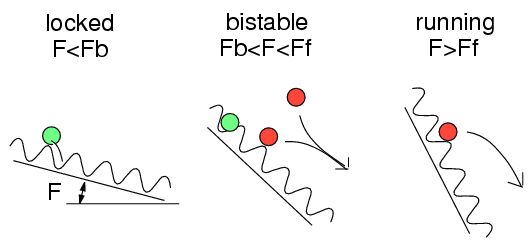One-dimensional models:

 Tomlinson model Frenkel-Kontorova model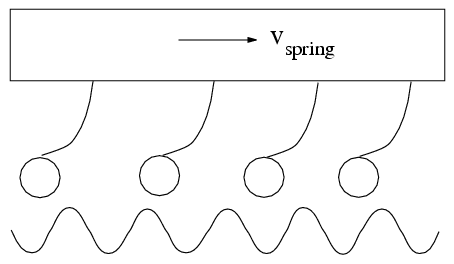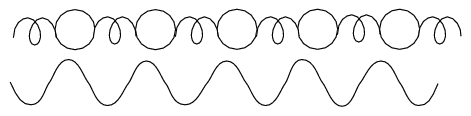(nice applets for the Tomlinson model and the dc driven FK model were written by F.-J. Elmer)

More complicated models:

• Tomlinson + FK (Elmer et al)

• Two FK chains (J.Röder et al)

• The FK chain between two external sinusoidal potentials (Rozman et al)

• Random substrate/springs (overdamped FK model, Cule and Hwa 1996) → directed percolation

Two-dimensional models

###Back to main page or tribology page

Last updated on October 15, 2008 by Oleg Braun.                    Translated from LATEX by TTH